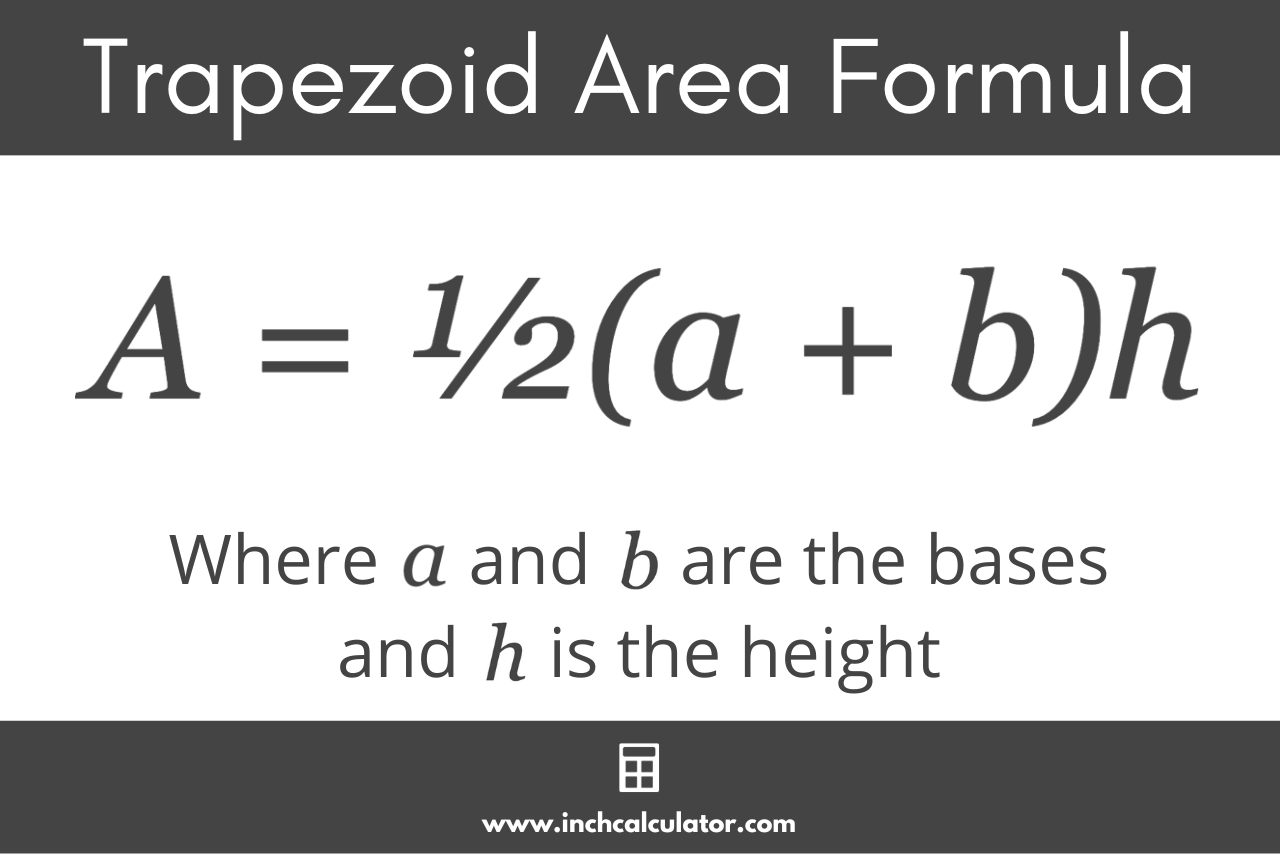# Trapezoid Area Calculator

Calculate the area of a trapezoid using the calculator below.## Area:

Learn how we calculated this below

## How to Calculate the Area of a Trapezoid

A trapezoid, also known as a trapezium in some parts of the world, is a four-sided figure with one pair of parallel sides called the bases. The other two sides, which are not parallel, are referred to as the legs.

The bases of a trapezoid are denoted a and b, while the legs are denoted c and d. The height is denoted h.You can calculate the area using the trapezoid area formula.

### Trapezoid Area Formula

The trapezoid area formula is:

A = ½(a + b)h

The area A of a trapezoid is equal to one-half of the sum of the length of the bases a and b, times the height h. This method finds the average of the bases and then uses the rectangle area formula to find the area by multiplying the average base length by the height.For example, let’s calculate the area of a trapezoid with bases of length 5 and 8 with a height of 4.

A = ½(5 + 8) × 4 = 26

So, this trapezoid has an area of 26.

You might also be interested in our parallelogram area calculator.